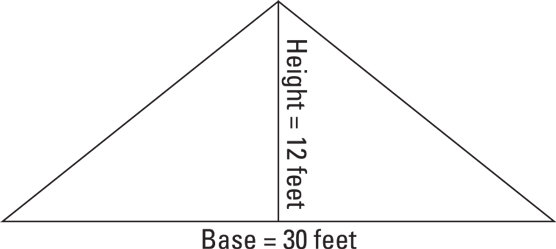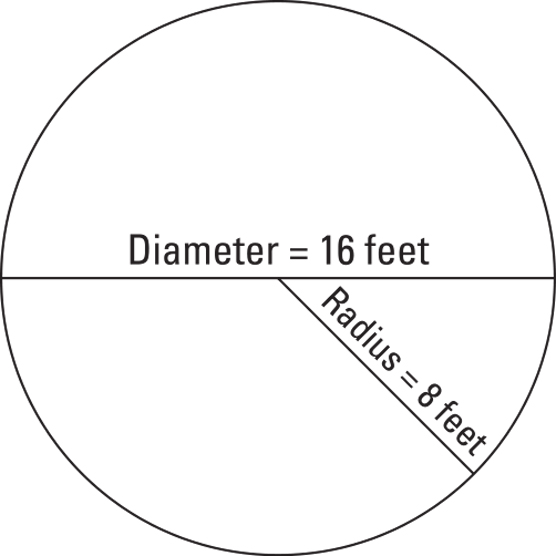##### Real Estate License Exams For DummiesBecause you'll be selling land and buildings as a real estate agent, for the real estate license exam, you need to know how to figure out what size they are. For the real estate license exam, all you really need to know is how to calculate the area and volume of land and buildings after you have the measurements.

Area is the amount of space on a flat surface. Calculating the area means figuring out how large a flat space is. It may be determining the square footage of a house, the amount of carpeting or tile needed to cover the floor of a room, or the amount of wallpaper needed to cover a wall. (Remember a wall still is a flat surface.)

Volume is the measurement of what it takes to fill up something. In real estate terms, calculating the volume of a warehouse, rather than just its floor area, is important when you're informing a potential buyer how much stackable space the property has.

## Calculating the Area of a Square or Rectangle

A square is a four-sided figure on which each side is the same length. A rectangle is a four-sided figure where two sides opposite each other are equal. For example, a rectangle may have two sides opposite each other that are 150 feet long, and the two other sides opposite each other are 80 feet long.

The formula for calculating the area of a square and a rectangle is the same.

Length (L) × Width (W) = Area (A)

Like most other calculations in this article, when calculating area, the units of measure must be the same. You have to multiply feet by feet, yards by yards, and so on. Furthermore, the answer to any area problem is always in square units. If you're multiplying feet by feet, your answer is in square feet.

You have a square piece of property that measures 100 feet by 100 feet. How big is the property?
100 feet × 100 feet = 10,000 square feet
Or, say you have a rectangular piece of property that measures 80 feet by 150 feet. What is the area?
150 feet × 80 feet = 12,000 square feet

Notice in this second question the longer figure is the length. It really doesn't matter in a problem like this what you call each number. On a piece of land, the distance across the front of the property generally is referred to as the width, while the distance going from the street back is the length or depth. Sometimes a problem may refer to a front foot or front footage, or frontage, which usually is the same as the width of the property along the street. If you get a problem that simply states two measurements of a lot, say 100 feet by 200 feet, assume that the first number is the measurement across the front of the lot, namely the frontage or width.

## Figuring Out the Area of a Triangle

A triangle is a three-sided figure where all the sides join together. Look up at the end of a peaked roofed house and you'll see a triangle. That triangle is called the gable end, and you may have to calculate the area to determine how much paint or siding is needed to cover it.

The formula for the area of a triangle is

1/2 Base (B) × Height (H) = Area (A)
Some of you may have learned this equation as
Base (B) × Height (H) / 2 = Area (A)Triangle with a base of 30 feet and a height of 12 feet.

Similar to the way you calculate the area of a square or rectangle, when calculating a triangle’s area, don’t forget that all the units of measurement must be the same and the answer must be in square units. The height is a line that is perpendicular, or at a right (90-degree) angle, to the base.

Say you want to paint your roof’s gable end. The width of the house at that point is 30 feet. The height of the gable is 12 feet. How many square feet are you going to have to paint?

0.5 × 30 feet × 12 feet = 180 square feet

## Calculating the Area of a Circle

A circle essentially is a single line that curves around and meets itself. Pick up that quarter in your pocket, and you’ve got a circle. The diameter is a straight line drawn through the center from one side of the circle to the other, and the radius is half of the diameter.

The formula to find the area of a circle is

π is the constant number 3.1416.Circle with a diameter of 16 feet.

Maybe you’re going to build a round patio. Or, if you live in farm country, you may have to rely on some of these calculations when determining the volume of a silo.

You’re building a circular patio that’s 16 feet in diameter. How many square feet of patio block will you use?

3.1416 × 8 (squared) = A (area)

3.1416 × (8 × 8) = 201.06 square feet

## Figuring Out the Area of an Irregular Shape

Unfortunately, many houses aren’t single squares or rectangles but rather are combinations of several squares and rectangles. A rectangular house with a small breezeway and attached garage is a good example of what I’m talking about. And the key to calculating the area of a house like this is that you have to divide the figure into squares and rectangles and occasionally even triangles, calculate the area of each individual figure, and then add them together. You can also come pretty close by using this technique to calculate the area of an irregular piece of land.

## Determining the Volume of Almost Anything

The formula for calculating the volume of any six-sided figure involves calculating the area and multiplying it by the height. Why six sided? Most shapes that you may encounter in your real estate work will have six sides with opposite sides of equal dimensions. Think of a warehouse (the example that follows) or a nice concrete patio in your back yard. The first part of the formula looks like you’re finding the area of a flat surface. In the case of volume however you also want to account for the depth or height of the structure so the formula looks like this:

Length (L) × Width (W) × Height (H) = Volume (V)

Say you want to calculate the volume of a rectangular warehouse that measures 100 feet by 200 feet by 15 feet high. The equation would look something like:

200 feet × 100 feet × 15 feet = 300,000 cubic feet

Length × width is the formula for calculating the area, but by multiplying that answer (called the product) by the third dimension, the height, you get the volume. Just like when you calculate area, the measurements must always be in the same units. With volume, the answer is always in cubic units. So, if all three measurements are in feet, the answer will be in cubic feet.

In the case of a triangular-shaped object like an attic, in which the triangle’s height already is used to calculate the area, you multiply the area by the length of the attic. The whole formula is

1/2 Base (B) × Height (H) × Length (L) = Volume (V)

Finally, suppose you need to find the volume of a cylindrical object like a silo on a farm. Once again, you just have to multiply the area of the silo’s base by the silo’s height.

If you had a silo 20 feet in diameter and 30 feet high, what is the volume?

π × radius squared (r2) × Height (H) = Volume (V)

3.1416 × (10 feet × 10 feet) × 30 feet= 9,424.8 cubic feet

Remember, the area of a circle involves the radius, which is half of the diameter. (That’s why this example uses 10 feet instead of 20 feet.)

In case you’re wondering about other shape figures, it's unlikely you'll have to deal with the volume of an octahedron (eight sides) or a tetrahedron (ten sides) or some other unusual shape building. The preceding formula should get you through the exam, your real estate career, and that patio you may want to build in your backyard.